# Multiplying and Dividing Positive and Negative Numbers

Author: Sophia Tutorial
##### Description:

Determine a product or quotient with positive and negative numbers.

(more)

Sophia’s self-paced online courses are a great way to save time and money as you earn credits eligible for transfer to many different colleges and universities.*

No credit card required

37 Sophia partners guarantee credit transfer.

299 Institutions have accepted or given pre-approval for credit transfer.

* The American Council on Education's College Credit Recommendation Service (ACE Credit®) has evaluated and recommended college credit for 32 of Sophia’s online courses. Many different colleges and universities consider ACE CREDIT recommendations in determining the applicability to their course and degree programs.

Tutorial
what's covered
1. Multiplying and Dividing Positive and Negative Numbers

# 1. Multiplying and Dividing Positive and Negative Numbers

Multiplication and division of integers both work in a very similar pattern to adding and subtracting. The short description of the process is that we multiply or divide like normal, and if the signs match (both positive or both negative) the answer is positive. If the signs don't match (one positive and one negative), the answer is negative.

Here are examples of multiplication and division of integers with matching signs: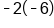Signs match, answer is positive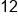Our Solution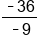Signs match, answer is positive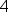Our Solution

In each example, since the two integers have matching signs, we multiply or divide the two numbers, and write the product or quotient as a positive number.

big idea
The product or quotient of two positive numbers is positive. The product or quotient of two negative numbers is also positive.

Here are some examples of multiplication and division of integers with opposite signs: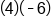Signs do not match, answer is negative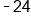Our Solution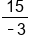Signs do not match, answer is negative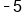Our Solution

In these examples, the two integers have opposite signs: one is positive and the other is negative. We multiply or divide the two numbers, and write the product or quotient as a negative numbers.

big idea
The product or quotient of a positive and negative number is negative.

hint
There are a few things to be careful of when working with integers. First, be sure not to confuse a problem like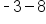with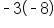.
The first problem,, is subtraction, because the subtraction separates -3 from what comes after it.
The second problem,, is a multiplication problem, because there is nothing between the 3 and the parenthesis. If there is no operation written in between the parts, then we assume that means we are multiplying.
Another item to watch out for is to be careful not to mix up the pattern for adding and subtracting integers with the pattern for multiplying and dividing integers. They can look very similar, for example if the signs match on addition, we keep the sign, even if it is negative: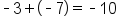. However, if the signs match in multiplication, the answer is always positive: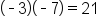.

summary
The big idea that multiplying or dividing by a negative number changes the sign of the product or quotient. When we multiply or divide two positive numbers, the answer is positive. When we multiply or divide by two negative numbers, the answer is also positive. However, when we multiply or divide a positive number and a negative number, the answer is negative.

Source: ADAPTED FROM "BEGINNING AND INTERMEDIATE ALGEBRA" BY TYLER WALLACE, AN OPEN SOURCE TEXTBOOK AVAILABLE AT: HTTP://WALLACE.CCFACULTY.ORG/BOOK/BOOK.HTML

Rating